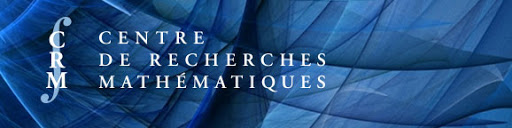# Preparatory Seminar

Welcome! This site is the homepage of the seminar series meant to prepare participants for the workshop on Higher Coleman Theory and its Applications to be held at the CRM (December 7-11, 2020).

Here is some material to prepare for the upcoming workshop (suggestions by Prof. Adrian Iovita):

## Background.

1) J.-P. Serre: A course in Arithmetic.

This is a good introduction to modular forms for people who didn’t see them.

2) Swinnerton-Dyer: $$\ell$$-Adic representations and congruences for coefficients of modular forms.

This paper has a chapter in which he defines modular forms modulo $$p$$.

3) J.-P. Serre: Formes modulaires et fonctions zeta $$p$$-adiques (French).

In this article, Serre defines $$p$$-adic modular forms as $$q$$-expansions.

4) N. Katz: $$p$$-Adic properties of modular forms and modular schemes.

This is an important, but long paper: he defines overconvergent modular forms, he constructs canonical subgroups of elliptic curves, finally he defines geometrically the $$p$$-adic modular forms of Serre.

Articles 2, 3, 4 can be found here

5) R. Coleman: $$p$$-Adic Banach spaces and families of modular forms.

This is an important paper but difficult to read. He defines the Coleman $$p$$-adic families of elliptic modular forms and $$q$$-expansions.

6) A. Ash, G. Stevens: $$p$$-Adic Deformation of Arithmetic Cohomology.

This paper has a chapter in which slope decomposition of a compact operator on a Banach module is explained very well.

7) V. Pilloni: Formes modulaires surconvergentes (French).

Here the Coleman families of elliptic modular forms are defined geometrically by first defining modular sheaves of arbitrary $$p$$-adic weight.

8) F. Andreatta, A. Iovita, V. Pilloni: $$p$$-Adic families of Siegel modular cuspforms.

Here the $$p$$-adic families of Siegel modular cuspforms are defined geometrically.

9) F. Andreatta, A. Iovita, V. Pilloni: $$p$$-Adic variation of automorphic sheaves.

In this paper the general machine of vector bundles with marked sections is constructed. This machine produces $$p$$-adic families of everything: modular sheaves, modular symbols, de Rham classes.

## Papers relevant for the workshop.

2) G. Boxer, V. Pilloni: Higher Hida and Coleman theory for modular curves.

3) D. Loeffler, V. Pilloni, C. Skinner, S. Zerbes: Higher Hida theory and $$p$$-adic $$L$$-functions for $$\rm {GSp}_4$$.

4) G. Boxer, V. Pilloni: Higher Coleman Theory

## Seminar Schedule

All talks will be in room 5340 (the amphitheater of 5-th floor. These talks will not be recorded)

Date/Time Speaker Topic
12 Nov, 10-11:30 Francesc Modular forms $$\pmod p$$ and $$p$$-adic modular forms. Hecke operators.
12 Nov, 13:30-15 Giovanni Katz modular forms. $$p$$-Adic modular forms viewed geometrically. Canonical subgroups of supersingular elliptic curves.
Some supplementary material
19 Nov, 10-11:30 Adrian Coleman families. Deformation of modular sheaves and Coleman families seen geometrically.
26 Nov, 10-11:30 Antonio C. Cohomology of coherent sheaves with support in a closed, on schemes and adic spaces.
26 Nov, 13:30-15 Ju-Feng Overconvergent Eichler-Shimura.
3 Dec, 14-15:30 Henri Euler systems and $$p$$-adic $$L$$-functions.
Some Supplementary material

Disclaimer: The attached hand-written notes were taken by yours truly during the lecture and may not be fully accurate.